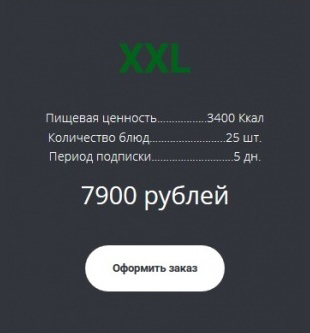# Официальная зарплата участников UFC Fight Night 120Опубликованы сведения по заработной плате бойцов-участников турнира UFC Fight Night 120.

Dustin Poirier (\$65,000 + \$65,000 за победу = \$130,000) - Anthony Pettis (\$135,000)

Matt Brown (\$75,000 + \$75,000 = \$150,000) - Diego Sanchez (\$95,000)

Andrei Arlovski (\$250,000) победи Junior Albini (\$12,000)

Cezar Ferreira (\$39,000 + \$39,000 = \$78,000) - Nate Marquardt (\$68,000)

Raphael Assuncao (\$56,600 + \$56,600 = \$113,200) - Matthew Lopez (\$26,400)

Clay Guida (\$57,000 + \$57,000 = \$114,000) - Joe Lauzon (\$62,000)

Marlon Moraes (\$70,000 + \$70,000 = \$140,000) - John Dodson (\$41,000)

Tatiana Suarez (\$25,000 + \$25,000 = \$50,000) - Viviane Pereira (\$14,000)

Sage Northcutt (\$60,000 + \$60,000 = \$120,000) - Michel Quinones (\$10,000)

Nina Ansaroff (\$18,000 + \$18,000 = \$36,000) - Angela Hill (\$21,000)

Sean Strickland (\$32,000 + \$32,000 = \$64,000) - Court McGee (\$35,000)

Jake Collier (\$18,000 + \$18,000 = \$36,000) - Marcel Fortuna (\$14,000)

Karl Roberson (\$10,000 + \$10,000 = \$20,000) - Darren Stewart (\$10,000)